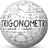# TrigonometryQuantitative Aptitude Questions and Answers section on “Trigonometry” with solution and explanation for competitive examinations such as CAT, MBA, SSC, Bank PO, Bank Clerical and other examinations.

# Aptitude Question ID : 94464

is equal to : [A] [B] [C] [D] Show Answer Hence option [C] is correct answer.

# Aptitude Question ID : 94460

If and are complementary to each other, then the value of is : [A]-1 [B]0 [C]1 [D]2 Show Answer 0 Hence option [B] is correct answer.

# Aptitude Question ID : 94453

If be an acute angle and then the value of is: [A] [B] [C] [D] Show Answer Hence option [B] is correct answer.

# Aptitude Question ID : 94449

The value of is : [A]-1 [B]1 [C] [D] Show Answer Hence option [C] is correct answer.

# Aptitude Questions : 94446

The angles of a triangle are and The value of x is : [A]28 [B]29 [C]30 [D]31 Show Answer 29 Sum of angles of a triangle Hence option [B] is correct answer.